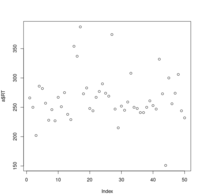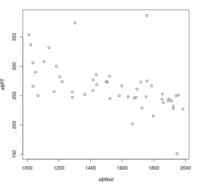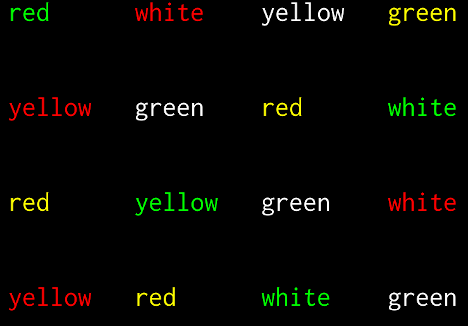# 12. Experiments¶

## 12.1. Simple reaction times¶

Many psychology experiments measure reaction-times or decision-times.

The script `simple-detection-visual-pygame.py` is a simple detection experiment programmed with pygame. The task is simple: the participant must press a key as quickly as possible when a cross appears at the center of the screen.

```python simple-detection-visual-pygame.py
```

The results are saved in `reaction_times.csv` which you can inspect with any text editor.

If you are an R afficionado, you can open it and type:

```data = read.csv('reaction_times.csv')
summary(data)
attach(data)
plot(RT)
dev.new()
plot(RT ~ Wait)
```

Here are my results:Simple Reaction Times as a function of trialRelationship between wait time and reaction time

Browse the code of `simple-detection-visual-pygame.py`

It is pretty technical! This is because Pygame is meant to program simple video games, not psychology experiments.

A more adequate library for this task is Expyriment (another one is Psychopy).

From here, we are going to use it to generate experiments.

Make sure you have installed Expyriment:

```.. code-block:: bash
```

\$ python >>> import expyriment

If an error message `moduleNotFoundError: No module named 'expyriment'` appears, check Software Installation.

Let us start by downloading `simple-detection-visual-expyriment.py` and run it with:

```python simple-detection-visual-expyriment.py
```

Then, in the subfolder `data`, locate a file with a name starting with `simple-detection...` and the extension `.xpd`. This is a text file containing the reactions times. To analyse them, download `analyse_rt.py` and run:

```python analyse_rt.py data/simple-detection-visual-expyriment_*.xpd
```

Compare the codes of `simple-detection-visual-expyriment.py` and `simple-detection-visual-pygame.py`. This should convince you that using expyriment will make your life simpler if you need to program a psychology experiment.

The documentation of expyriment is available at http://docs.expyriment.org/. Have q a quick look at it, especially http://docs.expyriment.org/expyriment.stimuli.html

The basic principles of the `expyriment` module are introduced in https://docs.expyriment.org/Tutorial.html. I provide a minimal template at `expyriment_minimal_template.py` that one can use to start writing a expyriment script.

Exercises:

1. Modify `simple-detection-visual-expyriment.py` to display a white disk instead of a cross.

2. Modify `simple-detection-visual-expyriment.py` to display a white disk on half of the trials and a gray disk on the other half of the trials (thesis experimental conditions should be shuffled randomly). Then modify it to display disks with four levels of gray. Thus you can assess the effect of luminosity on detection time. (see `xpy_simple_reaction_times/grey-levels.py` for a solution using Expyriment’s `design.Block` and `design.Trial` objects).

3. Modify `simple-detection-visual-expyriment.py` to play a short sound (`click.wav`) in lieu of displaying a visual stimulus (hint: use `stimuli.Audio()`). Thus, you have created a simple audio detection experiment.

4. Download and run `simple-detection-audiovisual.py`:

```python simple-detection-audiovisual.py
```

There are three blocks of trials: a first one in which the target is always visual, a second one in which it is always a sound, and a third one in which the stimulus is, randomly, visual or auditory. Are we slowed down in the latter condition? Use `analyse_audiovisual_rt.py` to analyse the reaction times.

Exercice: add python code to `simple-detection-audiovisual.py` to display instructions at the start of the experiment.

## 12.2. Decision times¶

In the previous example, the user just had to react to a stimulus. This involved a very simple type of decision (“is a target present or not?”)

Other tasks involves taking a decision about some property of the stimulus.

Exercise: | - Modify `simple-detection-visual-expyriment.py` to display, rather than a cross, a random integer between 0 and 9 (hint: Use `stimuli.TextLine()`). Now, the task is to decide if the figure is odd or even, by pressing one of two keys.

Here is a solution: `parity.py`
Comparing the average decision time to the time to react to a simple cross provides a (rough) estimate of the time to decide about the parity of a number. By the way, one can wonder what happens for multiple digits numbers: are we influenced by the flanking digits?
- Add feedback; when the subjects presses the wrong key, play the sound `wrong-answer.ogg`.

## 12.3. Numerical distance effect¶

Exercise: Create a script to present, at each trial, a random number between 1 and 99, and ask the subject to decide wether the presented number is smaller or larger than `55`. Plot the reactions times as a function of the number.

Do you replicate the distance effect reported by Dehaene, S., Dupoux, E., & Mehler, J. (1990) in “Is numerical comparison digital? Analogical and symbolic effects in two-digit number comparison.” Journal of Experimental Psychology: Human Perception and Performance, 16, 626–641.?

## 12.4. Lexical Decision¶

In a lexical decision experiment, a string of characters is flashed at the center of the screen and the participant has to decide if it is a actual word or not, indicating his/her decision by pressing a left or right button. Reaction time is measured from the word onset, providing an estimate of the speed of word recognition.

Let us program such a task.

### 12.4.1. Step 1: stimuli listed in constants¶

Modify the `parity task script` to display either a word or a pseudoword at each trial (in a random order).

For testing purposes, you can use the following variables:

```words = ['bonjour', 'chien', 'président']
pseudos = ['lopadol', 'mirance', 'clapour' ]
```

A solution is proposed in `lexdec_v1.py`

### 12.4.2. Step 2: read stimuli from a csv file¶

Then modify the lexical decision script to read the stimuli from a comma-separated text file (stimuli.csv) with two columns. Here is the content of `stimuli.csv`:

```item,category
bonjour,W
chien,W
président,W
mirance,P
clapour,P
```

(hint: To read a csv file, one can use `pandas.read_csv()`)

A solution is proposed in `lexdec_v2.py`

Note: You can use a file comparator, e.g. meld, to compare the two versions:

```meld lexdec_v1.py lexcdec_v2.py
```

### 12.4.3. Select words in a lexical dabatase¶

1. Click on “Recherche en Ligne” and play with the interface:

• enter `5...5` in the `nbletters` field

• enter `^b.t\$` in the field `ortho` field (see http://www.lexique.org/?page_id=101 for more examples of pattern that can be used)

2. how many words of grammatical category (`cgram`) ‘NOM’, and of length 5 (`nblettres`), of lexical frequency (`freqfilms2`) comprised between 10 and 100 per millions are there in this database? (answer=367). Save these words (i.e. the content of the field `Words`) into a `words.csv` file (you may have to clean manually, ie. remove unwanted columns, using Excel or Libroffice Calc).

### 12.4.4. Automatising database searches with R and Python¶

To select words, rather than using the interface at http://www.lexique.org, one can write scripts in R or Python. This promotes reproducible science.

1. Open https://github.com/chrplr/openlexicon/tree/master/documents/Interroger-Lexique-avec-R and follow the instructions in the document `interroger-lexique-avec-R.pdf`

To select 100 five letters long nouns for our lexical decision, execute:

```import pandas
subset = lex.loc[(lex.nblettres == 5) & (lex.cgram == "NOM") & (lex.freqfilms2 > 10) & (lex.nombre == 's')]
samp = subset.sample(100)
samp2 = samp.rename(columns = {'ortho':'item'})
samp2.item.to_csv('words.csv', index=False)
```

This creates `words.csv`.

### 12.4.5. Generate nonwords¶

1. Write a function that returns a nonword (a string containing random characters)

```def pseudo(length):
""" returns a nonword of length `length` """
```

Solution at `create_nonwords.py`

2. Use this function to create a list of 100 nonwords and save it in a file `"pseudowords.csv"` (one pseudoword per line) (see https://www.pythontutorial.net/python-basics/python-write-text-file/)

### 12.4.6. Create a stimuli file¶

Merge `words.csv` and `pseudowords.csv` into a single `stimuli2.csv` file:

```import pandas
w['category'] = 'W'
p['category'] = 'P'
allstims = pandas.concat([w, p])
allstims.to_csv('stimuli2.csv', index=False)
```

### 12.4.7. Use sys.argv to pass the name of the file containing the list of stimuli¶

Modify lexdec_v2.py to be able to pass the name of the stimuli file as an argument on the command line:

```python lexdec_v3.py stimuli2.csv
```

(hint: use sys.argv[]; see https://www.geeksforgeeks.org/how-to-use-sys-argv-in-python/)

Solution at `lexdec_v3.py`

### 12.4.8. Improving the pseudowords¶

1. Check out the pseudoword generator UniPseudo

2. Generate a new list of pseudowords and add them to a new `stimuli3.csv` file

### 12.4.9. Data analysis¶

After running:

```python lexdec_v3.py stimuli2.csv
```

the subject’s responses are stored in the subfolder `data/` contains a file `lexdec...xpd`

You can download this `xpd file` as an example.

1. Use `pandas.read_csv(..., comment='#')` to read the responses into a pandas dataframe.

2. Compute the average reaction times for words and for pseudo-words.

3. Plot the distribution of reactions times using `seaborn.boxplot()`

4. Use `scipy.stats.ttest_ind()` to perform a Student t-test compairn gthe RTs of Words and Non-Words.

Check a solution `analyze_RT.py`

### 12.4.10. Auditory Lexical Decision¶

Transform `lexdec_v1.py` into an auditory lexical decision script using the sound files from the lexical decision folder <../experiments/xpy_lexical_decision/>:

```bonjour.wav
chien.wav
président.wav
clapour.wav
mirance.wav
```

Solution at `lexdec_audio.py`

### 12.4.11. Finally¶

Check out an example of a “real” lexical decision experiment at https://chrplr.github.io/PCBS-LexicalDecision

## 12.6. Stroop Effect¶

The Stroop effect (Stroop, John Ridley (1935). “Studies of interference in serial verbal reactions”. Journal of Experimental Psychology. 18 (6): 643–662. doi:10.1037/h0054651) may be the most well known psychology experiment. Naming the color of the ink is difficult when there is a confict with the word itself. This is interpreted as a proof that reading is automatic, i.e. cannot be inhibited.

In the previous chapter, we created Stroop cards with Pygame.Stroop card

Download `stroop.zip`. Extract the files and run:

```python stroop_task.py
```

The times are in the subfolder `data`. Compute the average reading times as a function of the language (using `R` or `python`).

Exercise: Program a Stroop task with a single colored word displayed at each trial. To record actual naming times, you will need to record (!) the subject’s vocal response. A simple solution is to run a audio recording application while the script is running. You script should play a brief sound each time you present a target. Then, with a audio editor (e.g. Audacity), you can locate the times of presentation of stimuli and the onsets of vocal responses. Check out the program “CheckVocal” at https://github.com/0avasns/CheckVocal which does just that!

## 12.7. A general audio visual stimulus presentation script¶

In some experiments, we know in advance the precise timing of all stimuli (the program flow does not depend on external events). A script that reads the timing of audiovisual stimuli in a csv file and presents them at the expected times is available at https://www.github.com/chrplr/audiovis

## 12.8. Sound-picture matching using a touchscreen¶

The `sentence-picture-matching.py` script presents a sound, followed by a picture and waits for the participant to press a button.

Exercise: Modify the previous script to present two pictures and use expyriment’s TouchScreenButtonBox to record the subject’s response, using the example from `expyriment/touchscreen_test/touchscreen-test.py`

## 12.9. More examples using Expyriment¶

Besides the examples from this course, you can find more expyriment scripts at